# RD Sharma Solutions for Class 8 Math Chapter 8 - Division of Algebraic Expressions (Part-1) Notes | Study RD Sharma Solutions for Class 8 Mathematics - Class 8

## Class 8: RD Sharma Solutions for Class 8 Math Chapter 8 - Division of Algebraic Expressions (Part-1) Notes | Study RD Sharma Solutions for Class 8 Mathematics - Class 8

The document RD Sharma Solutions for Class 8 Math Chapter 8 - Division of Algebraic Expressions (Part-1) Notes | Study RD Sharma Solutions for Class 8 Mathematics - Class 8 is a part of the Class 8 Course RD Sharma Solutions for Class 8 Mathematics.
All you need of Class 8 at this link: Class 8

#### Question 1: Write the degree of each of the following polynomials.(i) 2x2 + 5x2 − 7(ii) 5x2 − 3x + 2(iii) 2x + x2 − 8(iv) 1/2 y7−12y6+48y5−1012y7-12y6+48y5-10(v) 3x3 + 1(vi) 5(vii) 20x3 + 12x2y2 − 10y2 + 20Answer 1:(i)  Correction  : It is 2x3+5x2−7  instead of 2x2+5x2−7.The degree of the polymonial  2x3+5x2−7 is 3.(ii) The degree of the polymonial 5x2−35x+2 is 2.(iii) The degree of the polymonial 2x+x2−8 is 2.(iv) The degree of the polymonial 12y7−12y6+48y5−10 is 7.(v) The degree of the polymonial 3x3+1 is 3.(vi) 5 is a constant polynomial and its degree is 0.(vii) The degree of the polymonial 20x3+12x2y2−10y2+20 is 4.Question 2: Which of the following expressions are not polynomials?(i) x2 + 2x−2 (ii)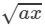+x2−x3(iii) 3y3 −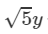5y + 9 (iv) ax1/2 + ax + 9x2 + 4(v) 3x−2 + 2x−1 + 4x +5 Answer 2: (i) x2+2x−2 is not a polynomial because −2 is the power of variable x is not a non negative integer. (ii)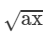+x2−x3 is not a polynomial because 1/2 is the power of variable x is not a non negative integer.

(iii) 3y3−√5y+9 is a polynomial because the powers of variable y are non negative integers.

(iv) ax1/2+ax+9x2+4 is not a polynomial because 1/2 is the power of variable x is not a non negative integer.
(v) 3x−2+2x−1+4x+5 is not a polynomial because −2 and −1 are the powers of variable x are not non negative integers.
Question 3: Write each of the following polynomials in the standard form. Also, write their degree.
(i) x2 + 3 + 6x + 5x4
(ii) a2 + 4 + 5a6
(iii) (x3 − 1)(x3 − 4)
(iv) (y3 − 2)(y3 + 11)
(v) (a3−8/3)(a3+16/17)
(vi) (a+3/4)(a+4/3)a+34a+43

####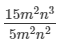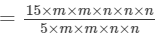=3m(22)n(32)=3m0n1=3n

####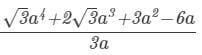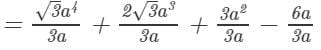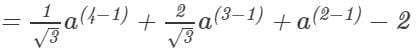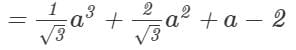The document RD Sharma Solutions for Class 8 Math Chapter 8 - Division of Algebraic Expressions (Part-1) Notes | Study RD Sharma Solutions for Class 8 Mathematics - Class 8 is a part of the Class 8 Course RD Sharma Solutions for Class 8 Mathematics.
All you need of Class 8 at this link: Class 8Use Code STAYHOME200 and get INR 200 additional OFF

## RD Sharma Solutions for Class 8 Mathematics

88 docs

Track your progress, build streaks, highlight & save important lessons and more!

,

,

,

,

,

,

,

,

,

,

,

,

,

,

,

,

,

,

,

,

,

;